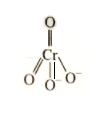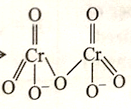Transition elements
Question

# The sum of the total number of bonds between chromium and oxygen atoms in chromate and dichromate ions is _____.

Moderate
Solution

## $\to$No. of bonds = 7 $\to$${\left({\mathrm{Cr}}_{2}{\mathrm{O}}_{7}\right)}^{2-}$No. of bonds = 12$⇒$Total number of Cr and O bonds is 19.

Get Instant Solutions